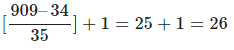Courses

# Arithmetic Progression Questions with Answers Quant Notes | EduRev

## CAT : Arithmetic Progression Questions with Answers Quant Notes | EduRev

The document Arithmetic Progression Questions with Answers Quant Notes | EduRev is a part of the CAT Course Quantitative Aptitude (Quant).
All you need of CAT at this link: CAT

Question 1:
In an arithmetic progression having 30 terms, the sum of 13 terms is equal to the sum of 27 terms. Which of the following is necessarily true?
a) The sum of all the terms in the progression is 0.
b) The sum of all the terms of the progression is negative.
c) 14th term of the progression is 0.
d) None of these
The sum of all the terms will not be zero. The sum of 13 terms is equal to that of 27 terms. So this means that the sum of the terms from 14th to 27th is 0. But this does not mean that sum of all the terms is 0.
The sum of all the terms can either be positive or negative. It will depend on whether the common difference is positive or negative.
14th term will not be 0.
Thus none of the given statements is necessarily true.
Hence option d is the correct answer.

Question 2:
Two arithmetic progressions are defined as below

A = {4 , 9 , 14 , 19 . . . . . . . . . .999}

B = {6, 13, 20, 27, . . . . . . . . . . 909}

If the set C = A∩B then how many subsets does C have?

a) 227

b) 225

c) 414

d) 413

The first common term of A and B is 34. The common difference of A is 5 and common difference of B is 7, so the next term common between the two progressions = 34 + LCM(5,7)
= 34 + 35 = 69
Hence the element of C will be 34, 69, 104 ,139 . . . .
Set B exhausts at 909 So number of elements in C =Hence, the number of subsets of C = 226 = 413

Question 3:
From the first 25 natural numbers, how many arithmetic progressions of 6 terms can be formed such that common difference of the AP is a factor of the 6th term.
a) 31
b) 32
c) 30
d) 28
Let the first term of the AP be a and the common difference be d.
The sixth term of the series will be a+5d
Given that d should be a factor of a+5d
⇒ a+5d is divisible by d
⇒ a should be divisible by d
So the required cases are
d = 1, a = 1,2,3…….20
d= 2 , a = 2,4,6…….14
d = 3, a = 3, 6,9
d= 4, a = 4
So the required number of AP’s are 20+7+3+1 = 31

Question 4:
The internal angles of a convex polygon are in arithmetic progression with a common difference of 10 degrees. If the smallest angle is 100 degrees, what is the number of sides of the polygon?
a) 10
b) 7
c) 8
d) 9
Let the number of sides of the polygon be n.
Hence, the sum of the internal angles of the polygon equals (n-2)*180.
The sum of the arithmetic progression equals n/2*(200 + (n-1)*10)
Hence, (n-2)*180 = n(100 + 5n – 5) = n(5n+95)
Therefore,
n2+19n = 36n–72
So, n2–17n+72 = 0
Hence,
n = 8
or
n = 9
If n = 9, the biggest angle becomes 100+(9-1)*10 = 180 degrees.
As this is not possible in a convex polygon, the correct answer is 8.

Question 5:
Find the number of common terms between the following two sequences
3,7,11, 15, 19 . . . . . .191
9, 14, 19, 24 . . . . . .224
a) 8
b) 9
c) 10
d) 7
Both the sequences are arithmetic progressions with a common difference of 4 and 5 respectively.
The first common term is 19. The next common term would occur after a difference of LCM (4,5) = 20. So the next common term would be 19 + 20 = 39. Similarly next would be 59 and so on. We can observe that first common term is 20*1 – 1, second is 20*2 -1 and so on. Since the first sequence lasts only till 191, the last common term will be less than 191.
The largest term of the form 20*n -1, less than 191 is 179 which can be written as 20*9 – 1.
Thus we can see that n varies from 1 to 9. Thus there are 9 common terms.

Offer running on EduRev: Apply code STAYHOME200 to get INR 200 off on our premium plan EduRev Infinity!

## Quantitative Aptitude (Quant)

116 videos|131 docs|131 tests

,

,

,

,

,

,

,

,

,

,

,

,

,

,

,

,

,

,

,

,

,

;